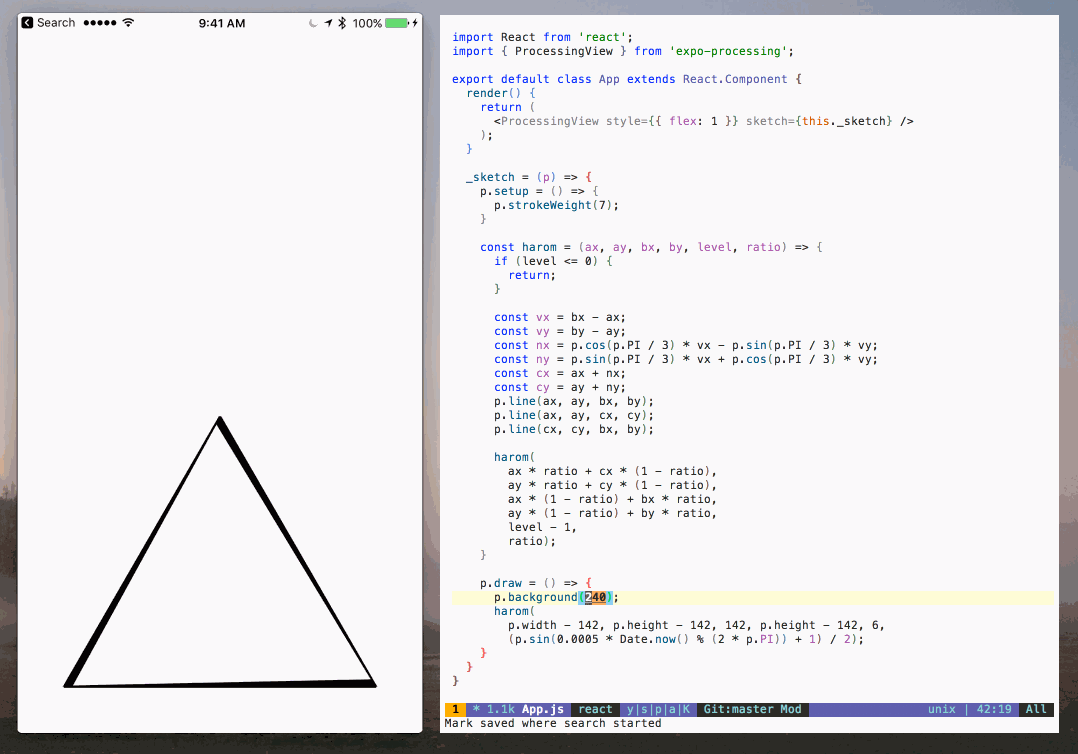expo-processing
Utilities for using Processing.js on ExpoLast updated 3 months ago by tsapeta .
MIT · Repository · Bugs · Original npm · Tarball · package.json
`\$ cnpm install expo-processing `
SYNC missed versions from official npm registry.NOTE: GIF above looks weird some times because it's a GIF, the real thing is nice, I promise...

# expo-processing

Use Processing.js on Expo! Just `npm i -S processing-js expo-processing` in your Expo project and import it with `import { ProcessingView } from 'expo-processing;`.

## Components

### `ExpoProcessing.ProcessingView`

Display a `Processing.js` sketch.

#### Props

The component accepts all `View` layout props for specifying its layout.

• `sketch`: A Processing.js sketch function that takes a `processing` instance and calls Processing.js functions on it, such as the `sketchProc` function in the Processing.js documentation for writing JavaScript-only Processing.js code.

## Example

This is based on the "In and out" sketch on OpenProcessing.org.

In a new blank Expo project, run `expo install processing-js expo-processing expo-gl` to install Processing.js and ExpoProcessing. Then replace `App.js` with the following:

``````import React from "react";
import { ProcessingView } from "expo-processing";

export default class App extends React.Component {
render() {
return <ProcessingView style={{ flex: 1 }} sketch={this._sketch} />;
}

_sketch = p => {
p.setup = () => {
p.strokeWeight(7);
};

const harom = (ax, ay, bx, by, level, ratio) => {
if (level <= 0) {
return;
}

const vx = bx - ax;
const vy = by - ay;
const nx = p.cos(p.PI / 3) * vx - p.sin(p.PI / 3) * vy;
const ny = p.sin(p.PI / 3) * vx + p.cos(p.PI / 3) * vy;
const cx = ax + nx;
const cy = ay + ny;
p.line(ax, ay, bx, by);
p.line(ax, ay, cx, cy);
p.line(cx, cy, bx, by);

harom(
ax * ratio + cx * (1 - ratio),
ay * ratio + cy * (1 - ratio),
ax * (1 - ratio) + bx * ratio,
ay * (1 - ratio) + by * ratio,
level - 1,
ratio
);
};

p.draw = () => {
p.background(240);
harom(
p.width - 142,
p.height - 142,
142,
p.height - 142,
6,
(p.sin((0.0005 * Date.now()) % (2 * p.PI)) + 1) / 2
);
};
};
}
``````

## Current Tags

• 2.0.2                                ...           latest (3 months ago)

## 7 Versions

• 2.0.2                                ...           3 months ago
• 2.0.1                                ...           6 months ago
• 2.0.0                                ...           6 months ago
• 1.0.3                                ...           3 years ago
• 1.0.2                                ...           3 years ago
• 1.0.1                                ...           3 years ago
• 1.0.0                                ...           3 years ago